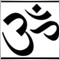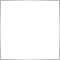# double OrderProfit( )24

Hi Dear

The function OrderProfit() returns the net profit of a selected trade, but the problem is that the profit is given in 3 options (As points, as deposit currency, term currency).

I think the value returned is the profit in deposit currency -i don't know what is term currency-, is there any solutions to have the profit as POINTS.

I need to know how much points are lost or winned in my positions, so please if you have any suggestions share it with me please.3196

`OrderOpenPrice() - OrderClosePrice()`24

qjol:
`OrderOpenPrice() - OrderClosePrice()`

This methode will give you information on closed oerders, i 'm talking about open trades5299

here's what i use, for example if i want the order to close every 20 pips of profit.

`if(OrderProfit()>Grid*OrderLots()*10){`

if OrderLots()=0.1, ----> 0.1*10 = 1 ----> if Grid=20 -----> 20*1=20

if OrderLots()= 1, -----> 1*10 = 10 ----> if Grid=20 -----> 20*10=200

So, humm now that I think about it .... if I wanna know how many pips that is... I'd need some devision.

`Pips=OrderProfit()/(OrderLots*10)`

This may or may not depend on the broker digits and/or pip value. I sucked at math in high school so mercy if my equation sucks lol.3196

razor_cell:

This methode will give you information on closed oerders, i 'm talking about open trades

(r u sure about that? LOL!!!) have you checked it out, try it & then talk to me5299

qjol:

(r u sure about that? LOL!!!) have you checked it out, try it & then talk to me

Yep, tested it and it works ... thanks qjol !!!1258

```int init ()                                                                                      //<   1>
{                                                                                                //<   2>
string acs.Operation [] = { "Buy" , "Sell"                                                   } ; //<   3>
//<   4>
double avd.QuotePoint   = MarketInfo  ( Symbol ()        , MODE_POINT       )                  ; //<   5>
double avd.QuoteTick    = MarketInfo  ( Symbol ()        , MODE_TICKSIZE    )                  ; //<   6>
double avd.NominalTick  = MarketInfo  ( Symbol ()        , MODE_TICKVALUE   )                  ; //<   7>
double ald.NominalPoint = avd.NominalTick                * avd.QuotePoint   / avd.QuoteTick    ; //<   8>
//<   9>
Alert ( ""                                                                                   ) ; //<  10>
Alert ( "avd.QuotePoint:   "          , DoubleToStr      ( avd.QuotePoint   , Digits       ) ) ; //<  11>
Alert ( "avd.QuoteTick:    "          , DoubleToStr      ( avd.QuoteTick    , Digits       ) ) ; //<  12>
Alert ( "avd.NominalTick:  "          , DoubleToStr      ( avd.NominalTick  , 2            ) ) ; //<  13>
Alert ( "ald.NominalPoint: "          , DoubleToStr      ( ald.NominalPoint , 2            ) ) ; //<  14>
//<  15>
if ( OrdersTotal ()   > 0             )                                                          //<  16>
{ int   i , N ; N  = OrdersTotal  () - 1                                                    ; //<  17>
for ( i = N ; i >= 0 ; i --      )                                                          //<  18>
{   OrderSelect    ( i         , SELECT_BY_POS    , MODE_TRADES      )                  ; //<  19>
//<  20>
double ald.OrderProfit     = OrderProfit      ()                                    ; //<  21>
double ald.ContractSize    = OrderLots        ()                                    ; //<  22>
double ald.OrderPoint      = ald.NominalPoint * ald.ContractSize                    ; //<  23>
int    ali.OrderProfit     = MathRound        ( ald.OrderProfit  / ald.OrderPoint ) ; //<  24>
//<  25>
Alert ( ""                                                                        ) ; //<  26>
//<  27>
Alert ( "Profit, points: " , ali.OrderProfit                                      ) ; //<  28>
//<  29>
Alert ( "Profit, "         , AccountCurrency  ()                                  ,   //<  30>
" : " , DoubleToStr      ( ald.OrderProfit  , 2            ) ) ; //<  31>
//<  32>
Alert ( "Order Point, "    , AccountCurrency  ()                                  ,   //<  33>
" : " , DoubleToStr      ( ald.OrderPoint   , 2            ) ) ; //<  34>
//<  35>
Alert ( "Size, lots:     " , DoubleToStr      ( ald.ContractSize , 2            ) ) ; //<  36>
Alert ( "Type:           " , acs.Operation    [ OrderType     () ]                ) ; //<  37>
Alert ( "Ticket:        #" , OrderTicket      ()                                  ) ; //<  38>
//<  39>
} // for                                                                                  //<  40>
} // if                                                                                       //<  41>
}                                                                                                //<  42>```3196

ubzen:

Yep, tested it and it works ... thanks qjol !!!

then next time don't...............24

OK, thank you very much for your time